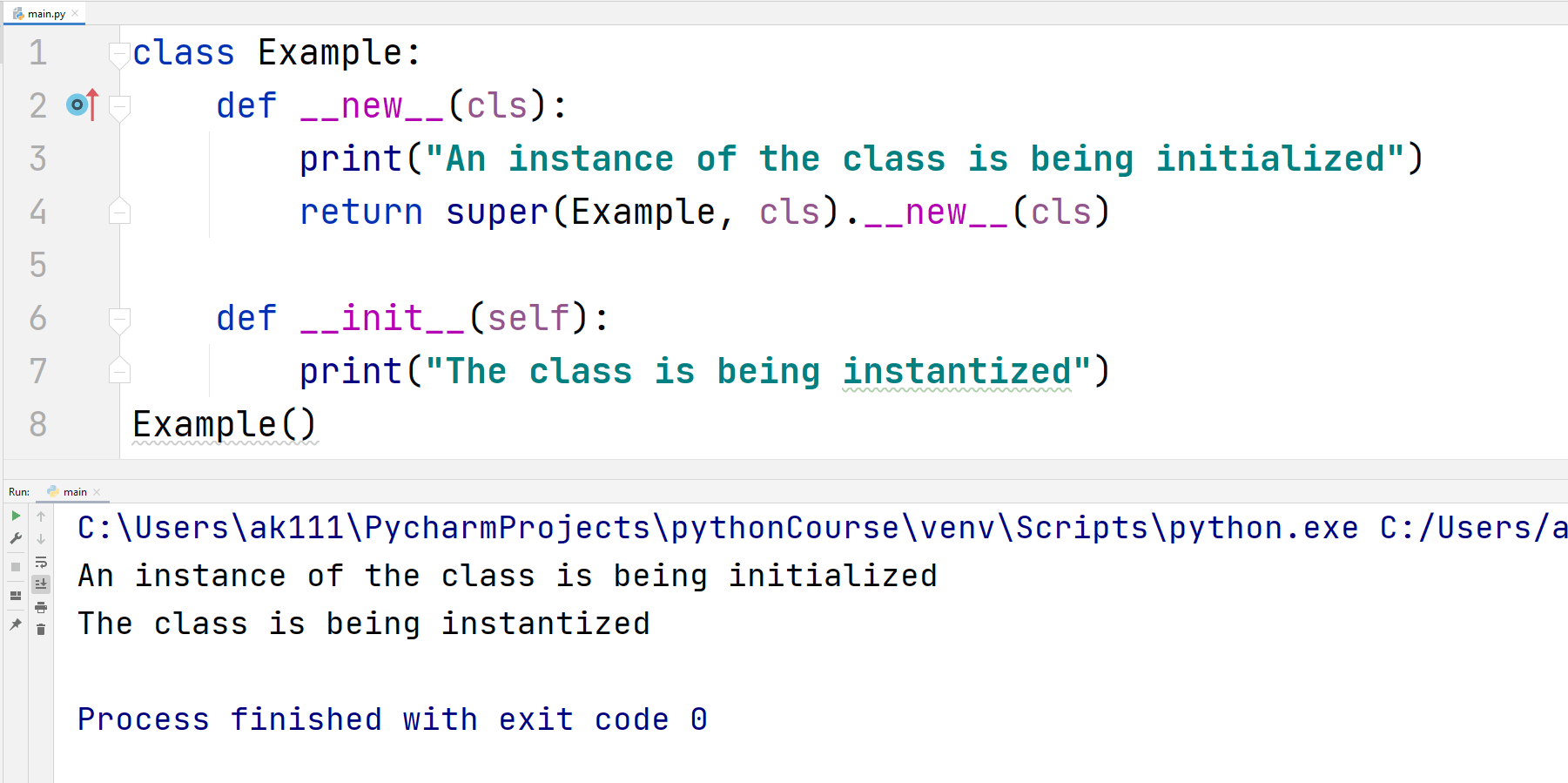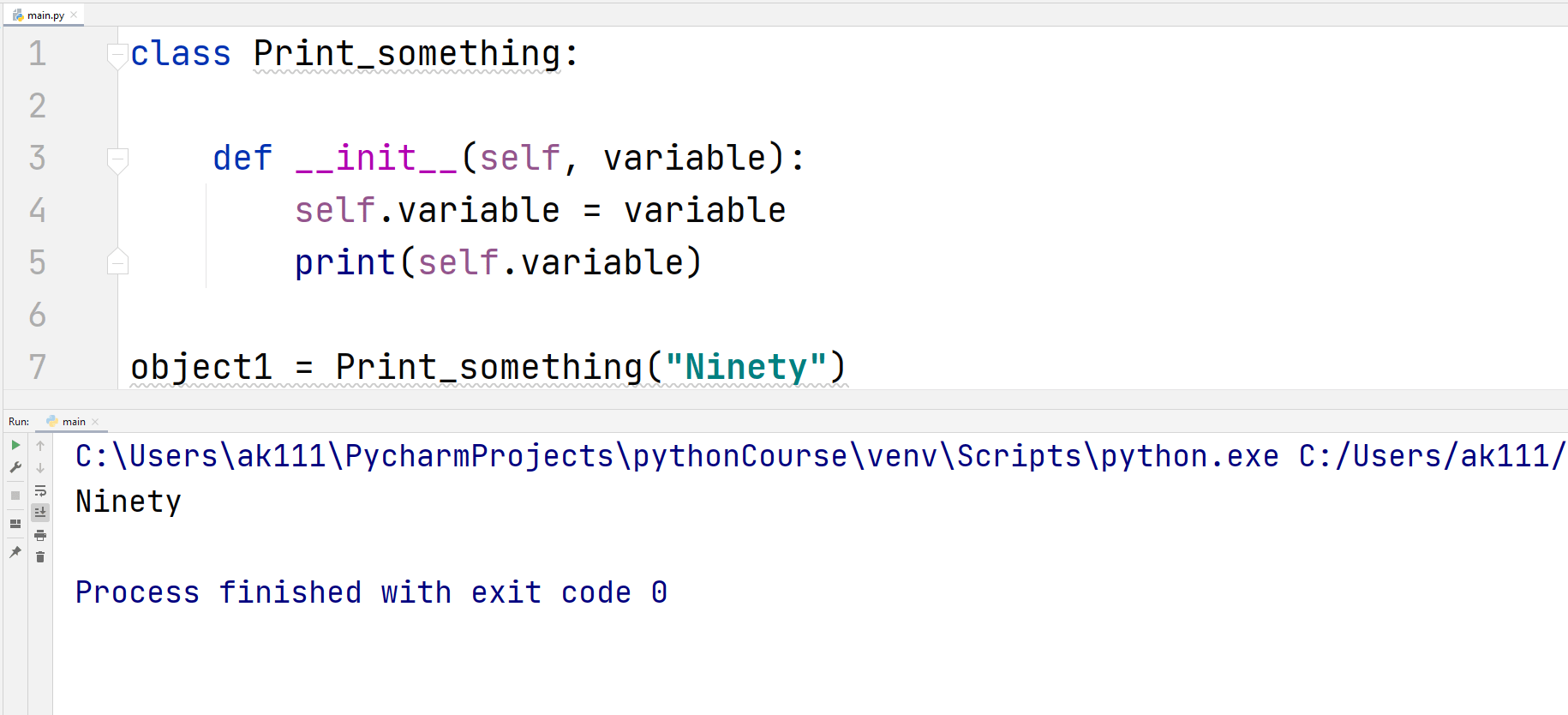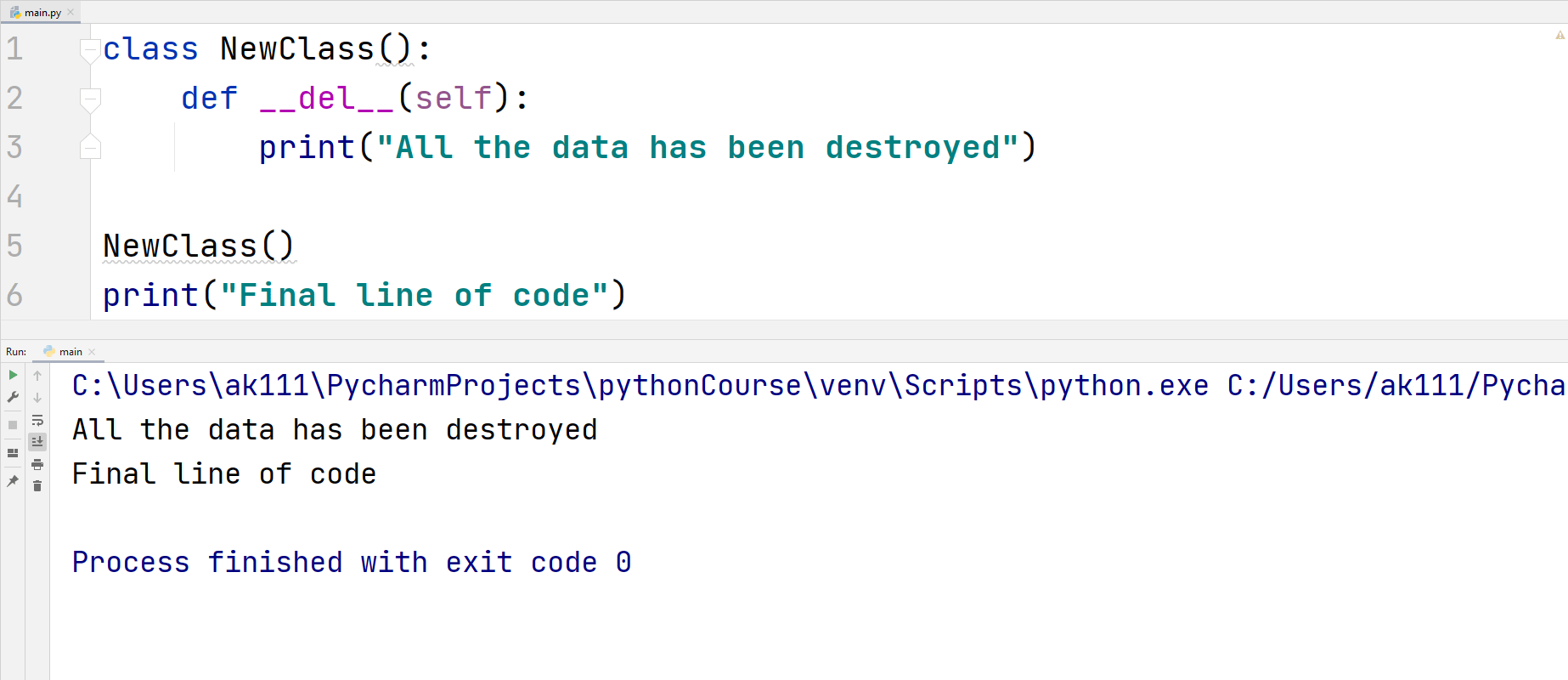# Python Magic Methods

## Python Magic Methods

No! Python might be better than many languages in many aspects but it cannot do wizardry. The magic methods in Python are the ones with two underscores on each side of the name. One example of Python magic methods is __init__().

The magic methods are pronounced as dunder method_name dunder, this is the reason magic methods are sometimes called dunder methods.

What is operator overloading? It is the ability of an operator to perform more than a single operation. For example, the ‘+’ operator can add two numbers or concatenate two strings together.

The reason why ‘+’ can add more than one string is because of these magic methods. Magic methods are the reason why Python is such a powerful language.

## Important Magic or Dunder Methods

Let’s try to understand important magic methods.

## __new__(cls, args)

When an object gets instantized, this is the first method that gets called. This is not used that much in Python. It is very useful when you want to subclass an immutable type like a tuple or a string.

__new__ is called when a new object is created. The __init__ method then initializes that object.

The __new__ method takes the class name and any arguments that the user has passed and then sends it to the __init__ method.

Syntax:

class Name_of_class:

def __new__(cls, *args, **kwargs):

return super(name_of_class, cls).__new__(cls, *args, **kwargs)

The super() method returns the parameters name_of_class, the cls object (a pointer to the class), your code statements and the arguments.

Let’s have an example to see the working of this dunder method.

## ExampleThe __init__ method will only get executed if __new__ returns something. So, if you remove the super() function from the code above, the __init__ method will not print anything.

## __init__(self, args)

The __init__() method acts as an initializer for the class. This receives all the arguments passed in the class parameters. It is used universally in Python classes because without it, a class will not get initialized.

In Python, the __init__ method is also called a constructor because it is always executed when objectifying a class. It’s main purpose is to initialize variables that we want to use with other functions.

Let’s have an example of this function.

## ExampleThe __init__ method cannot return anything. If you try to do that, Python will raise an error. But what type of error, let’s check with the below example.

## ExampleThis is because the purpose of __init__ method is to alter the state of the new instance. It cannot return anything except None.

## __repr__

The __repr__ magic method in Python is used to represent an object in class as a string. And, like the dunder methods used above, you can tweak representation of a string according to your choice.

This method is generally used for debugging the code. Let’s see an example for this function.

## ExampleCan you add a string in the output then? Well, let’s see if we can do that with the help of the following example.

## ExampleAs you can see, that did not work because they are of different types. To add this functionality in our class, we are going to use another magic method named __add__.

The __add__ magic method for the plus ‘+‘ operator. We can use this method to add a new string to our New_class type object in the output.

To do that, we are going to use a simpler example to avoid making the code longer.

## ExampleFor other mathematics operations like subtraction, division, etc., there are magic methods, for example, __sub__, __div__, etc.

### __del__

The __del__ magic method is a finalizer method. Python automatically calls it when the Python’s garbage collector is working.

The garbage in Python is collected when all the references to the class object have been removed. Or in simpler terms, this function is called when a function or code in a class has finished executing.

Let’s have an example of working of this function.

## ExampleWhen we create an instance of our NewClass() without assigning it to a variable, Python’s garbage collector keeps track of it.

Then, when the final line of code, i.e., the print statement is executed, the Python’s garbage collector deletes the NewClass() object entirely.

## List of Other Important Magic/Dunder Methods

### Augmented Assignment Methods

 Magic Method Method Description __iadd__(self, other) When the addition assignment x+=y is required, this method gets called. __imul__(self, other) This method gets called when the multiplication assignment x*=y is required. __isub__(self, other) This method gets called for the subtraction assignment x-=y. __idiv__(self, other) When the division assignment x/=y is required, this method gets called. __itruediv__(self, other) Gets called upon when true division is required. __imod__(self, other) This method gets called for the modulo assignment a%=b. __ipow__(self, other) The power calculation operator is **. When the exponent assignment a**=b is required, this method is used. __ifloordiv__(self, other) When the floor division assignment a//=b is required, this method gets called. __ilshift__(self, other) This method gets called when the left bitwise shift assignment a <<= b is required. __irshift__(self, other) This method gets called when the right bitwise shift assignment a >>= b is required. __ixor__(self, other) This dunder method is called when the bitwise XOR assignment a^=b happens. __ior__(self, other) This magic method gets called when the OR bitwise assignment operation a|=b happens. __iand__(self, other) This magic method gets called when the AND bitwise assignment operation a &= b happens.

### Type Conversion Methods

 Magic Method Method Description __int__(self) If you are converting an object to the type int, this method gets called. The method int() calls this function. __float__(self) When converting an object to float type, this method gets called by the float() method. __complex__(self) Called by the complex() method when Python converts an object to the complex type. __oct__(self) When you are creating an object type to octal, this method gets called by the oct() method. __hex__(self) When you are creating an object type to hexadecimal, this method gets called by the hex() method. __trunc__(self) The trunc() method from the math module calls this function. __index__(self) This dunder method is called when the object goes through a slicing operation.

### Unary Function and Operators

 Magic Method Method Description __abs__(self) This method is called by the abs() function. __pos__(self) Gets called when you perform the unary positive operation, +x. __neg__(self) Gets called when you perform the unary negative operation, -x. __invert__(self) The ~ operator is used for inversion. When you are doing inversion, this method gets called. __ceil__(self) Gets called when Python is executing the math.ceil() function. __floor__(self) Gets called when Python is executing the math.floor() function. __round__(self, n) When the built-in function round() is executed, this dunder method gets called.

### Operator Magic Methods

 Magic Method Method Description __add__(self, other) Gets called when the addition + operator is used. __sub__(self, other) Gets called when the subtraction – operator is used. __mul__(self, other) Gets called when the multiplication * operator is used. __truediv__(self, other) Gets called when the division/operator is used. __floordiv__(self, other) When using the // operator, this method gets called. __pow__(self, other) When using the ** operator, this method gets called. __mod__(self, other) Gets called when the mod % operator is used. __le__(self, other) This magic method gets called when you use the <= operator. __lt__(self, other) Gets called when performing operations with < operator. __eq__(self, other) When double equal signs == are used, this method gets called. __ge__(self, other) This magic method gets called when you use the >= operator. __ne__(self, other) When the not-equal sign != is used, this method gets called.

### String Magic Methods

 Magic Method Method Description __str__(self) When you use the str() method to convert something to string type, this dunder method gets used. __unicode__(self) Gets called when the unicode() method gets executed. __format__(self, formatstr) When you are formatting a string using the format() method, this dunder method gets called. __hash__(self) This dunder method returns an integer when you use the hash() method. __nonzero__(self) Returns True or False when the bool() method gets executed. __dir__(self) Used to return a list of attributes of a class when the dir() method is used. __sizeof__(self) Gets called when the getsizeof() method is called.

### Attribute Magic Methods

 Magic Method Method Description __getattr__(self, name) This method gets called when you are trying to access a non-existent attribute of a class. __setattr__(self, name) When you assign a value to the attribute of a class, this method gets called. __delattr__(self, name) Gets called when deleting an attribute of a class.